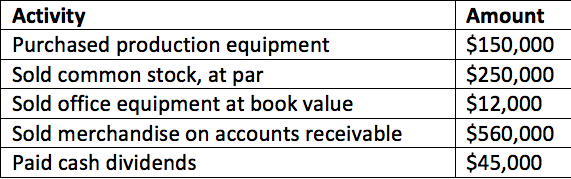# Problem: Consider the following: What was the net cash inflow (outflow) from investing activities? A. $138,000 B.$12,000 C. $183,000 D.$103,000

###### Problem Details

Consider the following:

What was the net cash inflow (outflow) from investing activities?

A. $138,000 B.$12,000

C. $183,000 D.$103,000Frequently Asked Questions

What scientific concept do you need to know in order to solve this problem?

Our tutors have indicated that to solve this problem you will need to apply the Investing Activities concept. You can view video lessons to learn Investing Activities. Or if you need more Investing Activities practice, you can also practice Investing Activities practice problems.

What is the difficulty of this problem?

Our tutors rated the difficulty ofConsider the following: What was the net cash inflow (outfl...as medium difficulty.